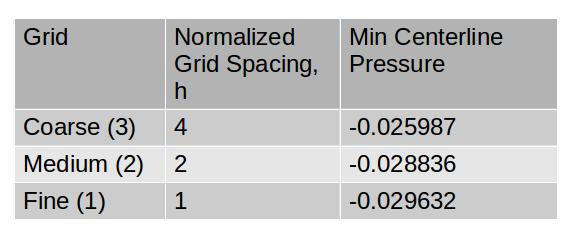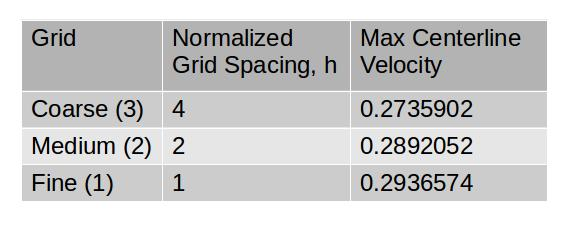Skip to content# Establishing Grid Convergence

Establishing grid convergence is a necessity in any numerical study. It is essential to verify that the equations are being solved correctly and that the solution is insensitive to the grid resolution. Some great background and details can be found from NASA:

https://www.grc.nasa.gov/WWW/wind/valid/tutorial/spatconv.html

First, here is a summary the equations and steps discussed here (in case you don’t want to read the whole example):

1. Complete at least 3 simulations (Coarse, medium, fine) with a constant refinement ratio, r, between them (in our example we use r=2)
2. Choose a parameter indicative of grid convergence. In most cases, this should be the parameter you are studying. ie if you are studying drag, you would use drag.
3. Calculate the order of convergence, p, using:
•$p=\ln(\frac{(f_3-f_2)}{(f_2-f_1)}) / \ln(r)$
4. Perform a Richardson extrapolation to predict the value at h=0
•$f_{h=0}=f_{fine}+\frac{f_1-f_2}{r^p-1}$
5. Calculate grid convergence index (GCI) for the medium and fine refinement levels
•$GCI=\frac{F_s |e|}{r^p-1}$
6. Ensure that grids are in the asymptotic range of convergence by checking:
•$\frac{GCI_{2,3}}{r^p \times GCI_{1,2}} \approxeq 1$

So what is a grid convergence study? Well, the gist of it is that you refine the mesh several times and compare the solutions to estimate the error from discretization. There are several strategies to do this. However, I have always been a fan of the following method: Create a very fine grid and simulate the flow problem. Then reduce the grid density twice, creating a medium grid, and coarse grid.

There are several strategies to do this. However, I have always been a fan of the following method: Create a very fine grid and simulate the flow problem. Then reduce the grid density twice, creating a medium grid, and coarse grid. To keep the process simple, the ratio of refinement should be the same for each step. ie. if you reduce the grid spacing by 2 in each direction between the fine and medium grid, you should reduce it again by 2 between the medium and coarse grid. In the current example, I generated three grids for the cavity problem with a refinement ratio of 2:

• Fine grid: 80 cells in each direction – (6400 cells)
• Medium grid: 40 cells in each direction – (1600 cells)
• Coarse grid: 20 cells in each direction – (400 cells)

Velocity contour plots are shown in the following figures:

We can see from the figures that the quality of the simulation improves as the grid is refined. However, the point of a grid convergence study is to quantify this improvement and to provide insight into the actual quality of the fine grid.

The accuracy of the fine grid is then examined by calculating the effective order of convergence, performing a Richardson extrapolation, and calculating the grid convergence index. As well, (as stated in the article from NASA), it is helpful to ensure that you are in the asymptotic range of convergence.

What are we examining?

It is very important at the start of a CFD study to know what you are going to do with the result. This is because different parameters will converge differently. For example, if you are studying a higher order parameter such as local wall friction, your grid requirements will probably be more strict than if you are studying an integral (and hence lower order) parameter such as coefficient of drag. You only need to ensure that the property or parameter that you are studying is grid independent. For example, if you were studying the pressure increase across a shockwave, you would not check that wall friction somewhere else in the simulation was converged (unless you were studying wall friction as well).  If you want to be able to analyze any property in a simulation, both high and low order, then you should do a very rigorous grid convergence study of primitive, integrated and derived variables.

In our test case lets pretend that in our research or engineering project that we are interested in the centerline pressure and velocity. In particular, let’s say we are interested in the profiles of these variables along the centerline as well as their peak values. The centerline profiles of velocity and pressure are shown in the following figures:

Calculate the effective order of convergence

From our simulations, we have generated the following data for minimum pressure along the centerline, and maximum velocity along the centerline:The order of convergence, p, is calculated with the following equation:$p=\ln(\frac{(f_3-f_2)}{(f_2-f_1)}) / \ln(r)$

where r is the ratio of refinement, and f1 to f3 are the results from each grid level.

Using the data we found, p = 1.84 for the minimum pressure and p=1.81 for the maximum velocity.

Perform Richardson extrapolation of the results

Once we have an effective order, p, we can do a Richardson extrapolation. This is an estimate of the true value of the parameter we are examining based on our order of convergence. The extrapolation can be performed with the following equation:$f_{h=0}=f_{fine}+\frac{f_1-f_2}{r^p-1}$

recall that r is the refinement ratio and is$h_2/h_1$ which in this case is 2.

Using this equation we get the Richardson extrapolated results:

•$P_{min}$ at h=0  -> -0.029941
•$V_{max}$ at h=o  -> 0.2954332

The results are plotted here:Calculate the Grid Convergence Index (GCI)

Grid convergence index is a standardized way to report grid convergence quality. It is calculated at refinement steps. Thus we will calculate a GCI for steps from grids 3 to 2, and from 2 to 1.

The equation to compute grid convergence index is:$GCI=\frac{F_s |e|}{r^p-1}$

where e is the error between the two grids and$F_s$ is an optional (but always recommended) safety factor.

Now we can calculate the grid convergence indices for the minimum pressure and maximum velocity.

Minimum pressure

•$GCI_{2,3} = 1.25 \times |\frac{-0.028836-(-0.025987)}{-0.028836}|/(2^{1.84}-1) \times 100 \% = 4.788 \%$
•$GCI_{1,2} = 1.25 \times |\frac{-0.029632-(-0.028836)}{-0.029632}|/(2^{1.84}-1) \times 100 \% = 1.302 \%$

Max velocity

•$GCI_{2,3} =1.25 \times | \frac{0.2892-0.27359}{0.2892}|/(2^{1.81}-1) \times 100 \% =2.69187 \%$
•$GCI_{1,2} = 1.25 \times |\frac{0.29365-0.2892}{0.29365}|/(2^{1.84}-1) \times 100 \% = 0.7559 \%$

Check that we are in the asymptotic range of convergence

It is also necessary to check that we are examing grid converegence within the asymptotic range of convergence. If we are not in the asymtotic range this means that we are not asymptotically approaching a converged answer and thus our solution is definitely not grid indipendent.

With three grids, this can be checked with the following relationship:$\frac{GCI_{2,3}}{r^p \times GCI_{1,2}} \approxeq 1$

If we are in the asymptotic range then the left-hand side of the above equation should be approximately equal to 1.

In our example we get:

Minimum Pressure$1.0276 \approxeq 1$

Minimum Velocity$1.0154 \approxeq 1$

Applying Richardson extrapolation to a range of data

Alternatively to choosing a single value like minimum pressure or maximum velocity. Richardson extrapolation can be applied to a range of data. For example, we can use the equation for Richardson extrapolation to estimate the entire profile of pressure and velocity along the centerline at h=0.

This is shown here:

In this post, we used the cavity tutorial from OpenFOAM to do a simple grid convergence study. We established an order of convergence, performed Richardson extrapolation, calculated grid convergence indices (GCI) and checked for the asymptotic range of convergence.

As I said before, the NASA resource is very helpful and covers a similar example:

As well the papers by Roache are excellent reading for anybody doing numerical analysis in fluids:

Categories

CFD from Scratch

Tags1.M M says:

Many thanks for the excellent demonstration.

I have a question which might be similar to someone’s else question. Does that mean you can use either of the three refinement level?

2.Jimmy says:

Can someone help me out here? When I try to use the order of convergence formula for the given data, I get p = 0.84 for the minimum pressure and p=0.81 for the maximum velocity. 1 less than the mentioned values. Am I missing something?

•curiosityFluidsAdmin1 says:

I’m not sure, I just checked and I get the same answers as in the page.

For pressure:

f3-f2=0.002849,
f2-f1=0.000796,
(f3-f2)/(f2-f1)=3.57915

LN(3.57915)/LN(2) = 1.84

•Jimmy says:

My bad, I was interpolating for grid spacing h=3 and using that value for f3. Thanks for the steps. Can I use grid spacings like 1,2,3,4… (arithmetic sequence instead of geometric sequence) for my own problem to calculate the order of convergence?

3.jounathanm says:

The lowest, I thik,
But it has to be said to what grade of refinement you refer

4.Ted says:

Excellent demonstration!

I have a question. After the cgi study, which grid should be used, fine or medium?

This site uses Akismet to reduce spam. Learn how your comment data is processed.